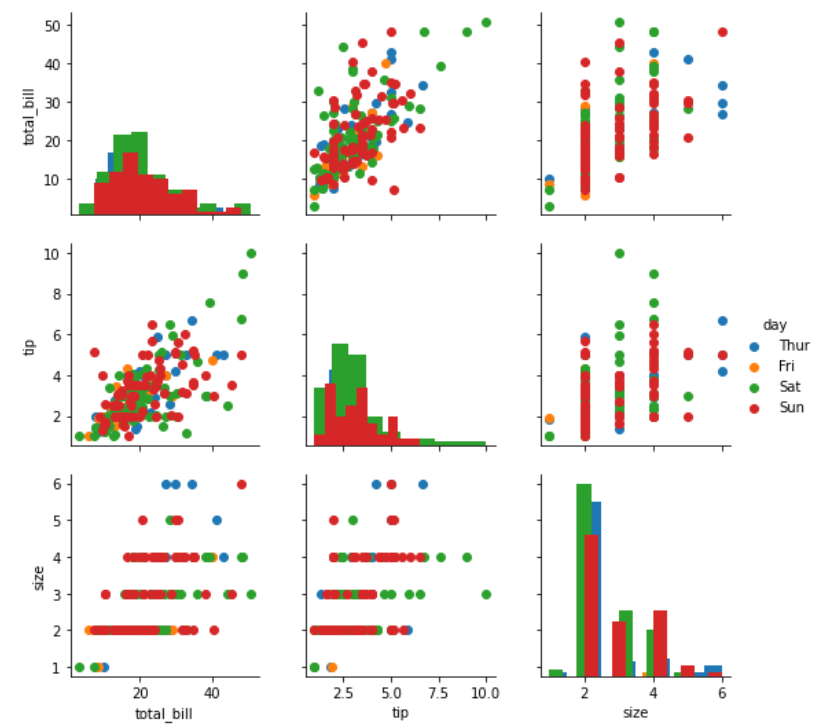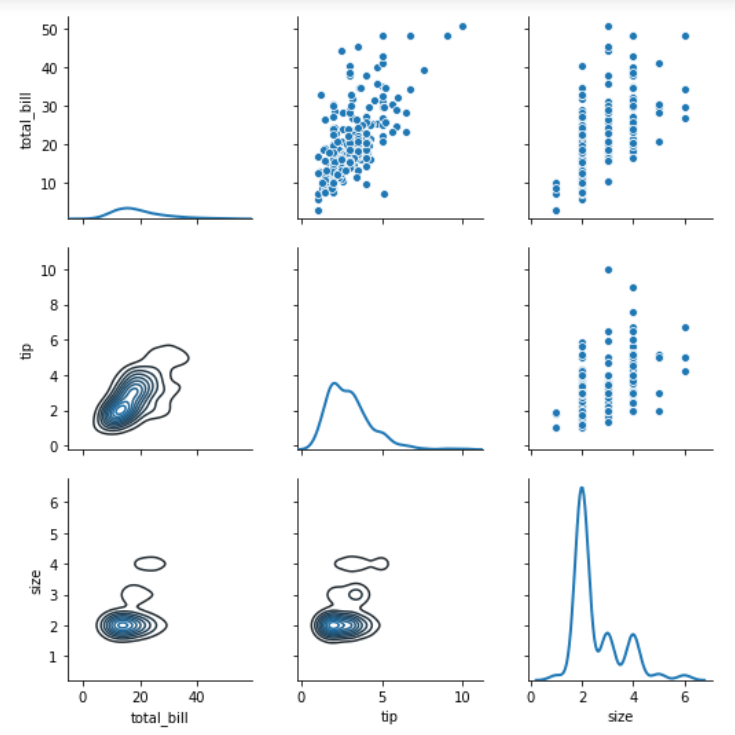# Python – seaborn.PairGrid() method

#### Prerequisite: Seaborn Programming Basics

Seaborn is a Python data visualization library based on matplotlib. It provides a high-level interface for drawing attractive and informative statistical graphics. Seaborn helps resolve the two major problems faced by Matplotlib; the problems are ?

• Default Matplotlib parameters
• Working with data frames

As Seaborn compliments and extends Matplotlib, the learning curve is quite gradual. If you know Matplotlib, you are already half way through Seaborn.

### seaborn.PairGrid() :

• Subplot grid for plotting pairwise relationships in a dataset.
• This class maps each variable in a dataset onto a column and row in a grid of multiple axes. Different axes-level plotting functions can be used to draw bivariate plots in the upper and lower triangles, and the the marginal distribution of each variable can be shown on the diagonal.
• It can also represent an additional level of conditionalization with the hue parameter, which plots different subsets of data in different colors. This uses color to resolve elements on a third dimension, but only draws subsets on top of each other and will not tailor the hue parameter for the specific visualization the way that axes-level functions that accept hue will.
```                    seaborn.PairGrid( data, \*\*kwargs)

```

Seaborn.PairGrid uses many arguments as input, main of which are described below in form of table:

Below is the implementation of above method:

Example 1:

## Python3

 `# importing packages ` `import` `seaborn ` `import` `matplotlib.pyplot as plt ` ` `  `# loading dataset ` `df ``=` `seaborn.load_dataset(``'tips'``) ` ` `  `# PairGrid object with hue ` `graph ``=` `seaborn.PairGrid(df, hue ``=``'day'``) ` `# type of graph for diagonal ` `graph ``=` `graph.map_diag(plt.hist) ` `# type of graph for non-diagonal ` `graph ``=` `graph.map_offdiag(plt.scatter) ` `# to add legends ` `graph ``=` `graph.add_legend() ` `# to show ` `plt.show() ` `# This code is contributed by Deepanshu Rusatgi.`

#### Output :## Python3

 `# importing packages ` `import` `seaborn ` `import` `matplotlib.pyplot as plt ` ` `  `# loading dataset ` `df ``=` `seaborn.load_dataset(``'tips'``) ` ` `  `# PairGrid object with hue ` `graph ``=` `seaborn.PairGrid(df) ` `# type of graph for non-diagonal(upper part) ` `graph ``=` `graph.map_upper(sns.scatterplot) ` `# type of graph for non-diagonal(lower part) ` `graph ``=` `graph.map_lower(sns.kdeplot) ` `# type of graph for diagonal ` `graph ``=` `graph.map_diag(sns.kdeplot, lw ``=` `2``) ` `# to show ` `plt.show() ` `# This code is contributed by Deepanshu Rusatgi.`

#### Output:My Personal Notes arrow_drop_upCheck out this Author's contributed articles.

If you like GeeksforGeeks and would like to contribute, you can also write an article using contribute.geeksforgeeks.org or mail your article to contribute@geeksforgeeks.org. See your article appearing on the GeeksforGeeks main page and help other Geeks.

Please Improve this article if you find anything incorrect by clicking on the "Improve Article" button below.

Article Tags :

Be the First to upvote.

Please write to us at contribute@geeksforgeeks.org to report any issue with the above content.